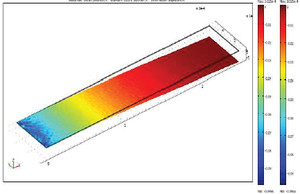# Articles techniques et présentations

#### Study of Effect on Resonance Frequency of Piezoelectric Unimorph Cantilever for Energy Harvesting

G. R. Prakash, K. M. V. Swamy, S. Huddar, B. G. Sheeparamatti, Kirankumar B. B.
Basaveshwar Engineering College, Bagalkot, Karnataka, India
Published in 2012

The focus of this paper is to study the effect on resonance frequency and power enhancement techniques of piezoelectric MEMS and modeling, design, and optimization of a piezoelectric generator based on a two-layer bending element(Figure 1) using COMSOL Multiphysics. An analytical relation was developed based on the shift in resonance frequency(Figure 2) caused by the addition of a thin film on the cantilever. The theoretical analysis is done with a user-friendly MATLAB/Simulink(Figure 3) interface constructed for easy introduction of design dimensions[2,3], material parameter values and force signal stimuli. The model has been validated and used not only to estimate power output under a given set of conditions, but also as the basis for generator design optimization. In addition to providing intuitive design insight, the system should be designed to resonate at the dominant driving frequency of the target vibrations if possible. Power output is proportional to the proof mass attached to the system. Therefore, the proof mass should be maximized while maintaining other constraints such as resonance frequency and strain limits. Power output is inversely related to the driving and resonance frequency. Therefore, designing for lower frequency peaks in the vibration range is preferred as long as they have equivalent or higher acceleration magnitude than higher frequency peaks[5,6]. Upon review of published piezoelectric microgenerators in this field, it is found that the proposed design of the piezoelectric energy harvester offers a best figure of merit (FOM) and promising output voltage(Figure 4).